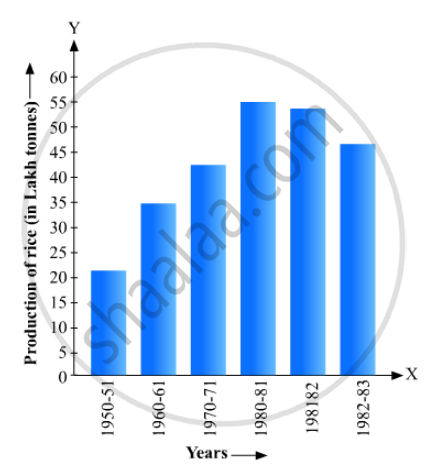# Read the Bar Graph Given in Fig. 23.17 and Answer the Following Questions: (I) What Information is Given by the Bar Graph? (Ii) What Was the Crop-production of Rice in 1970 - 71? - Mathematics

Read the bar graph given in Fig. 23.17 and answer the following questions:
(i) What information is given by the bar graph?
(ii) What was the crop-production of rice in 1970 - 71?
(iii) What is the difference between the maximum and minimum production of rice?#### Solution

(1) The bar graph represents the production of rice crop in India in different years.

(2) According to the height of the 3rd bar from the left, the crop-production of rice in 1970-71 is 42.5 lakh tonnes.

(3) The maximum product of rice is 55 lakh tonnes (height of the 4th bar from the left) in the year 1980-81 and the minimum product of rice is 22 lakh tonnes (height of the 1st bar from the left) in the year 1950-51. Hence, the difference between maximum and minimum production of rice (in lakh tonnes) is = 55 – 22 = 33 .

Concept: Graphical Representation of Data
Is there an error in this question or solution?

#### APPEARS IN

RD Sharma Mathematics for Class 9
Chapter 23 Graphical Representation of Statistical Data
Exercise 23.1 | Q 13 | Page 14

Share